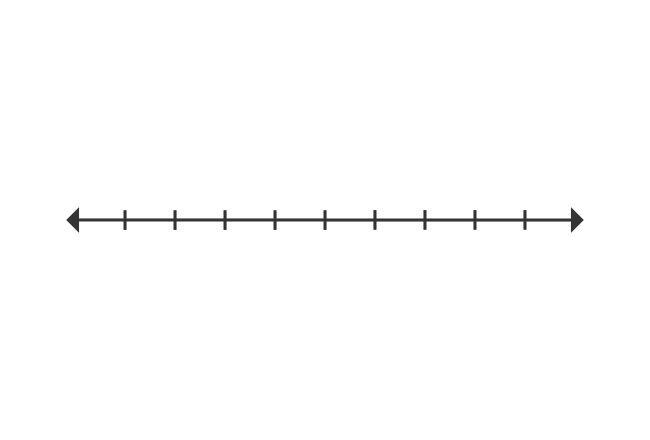# Number Line

A straight-line whose length splits as the required number of equal parts is called a number line.

## IntroductionA number line is basically a straight line but its length is divided as required number of equal parts and each division is represented by a real number.

The arrow heads at both sides of the number line indicate that the line and real numbers can be extended up to infinity at both sides.

It is basically used to identify the location of any point on the plane by comparison. It is also used to understand the arithmetic operations.

### Construction

A number line can be drawn geometrically in four simple steps.1. Draw a straight line of any length.
2. Divide the length of the straight line as required number of equal parts by marking points.
3. Identity centre point and represent it by zero.
4. Represent the points, right side to the centre by positive real numbers and also represent the points, left side to the centre by negative real numbers.
Latest Math Topics
Jun 26, 2023
Jun 23, 2023

Latest Math Problems
Jul 01, 2023
Jun 25, 2023
###### Math Questions

The math problems with solutions to learn how to solve a problem.

Learn solutions

Practice now

###### Math Videos

The math videos tutorials with visual graphics to learn every concept.

Watch now

###### Subscribe us

Get the latest math updates from the Math Doubts by subscribing us.